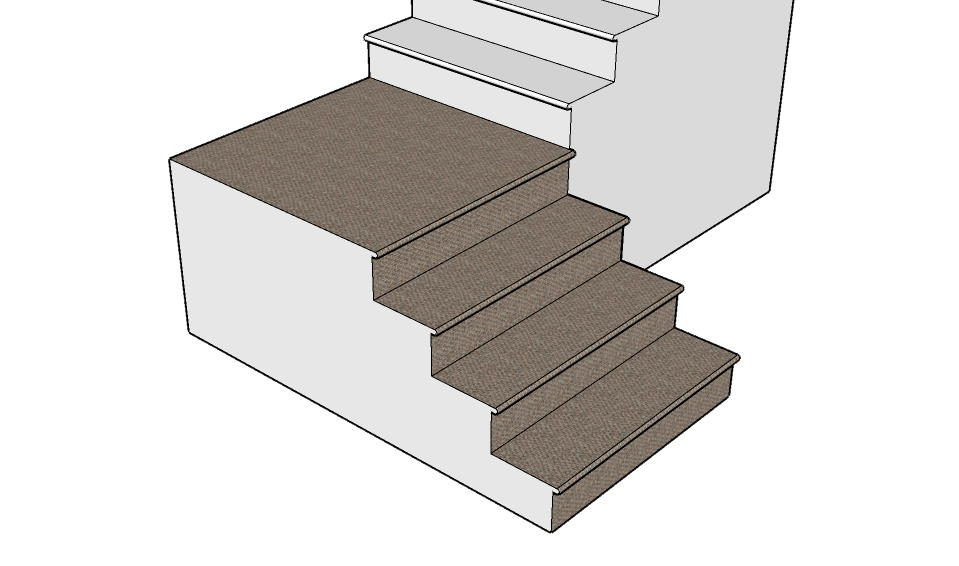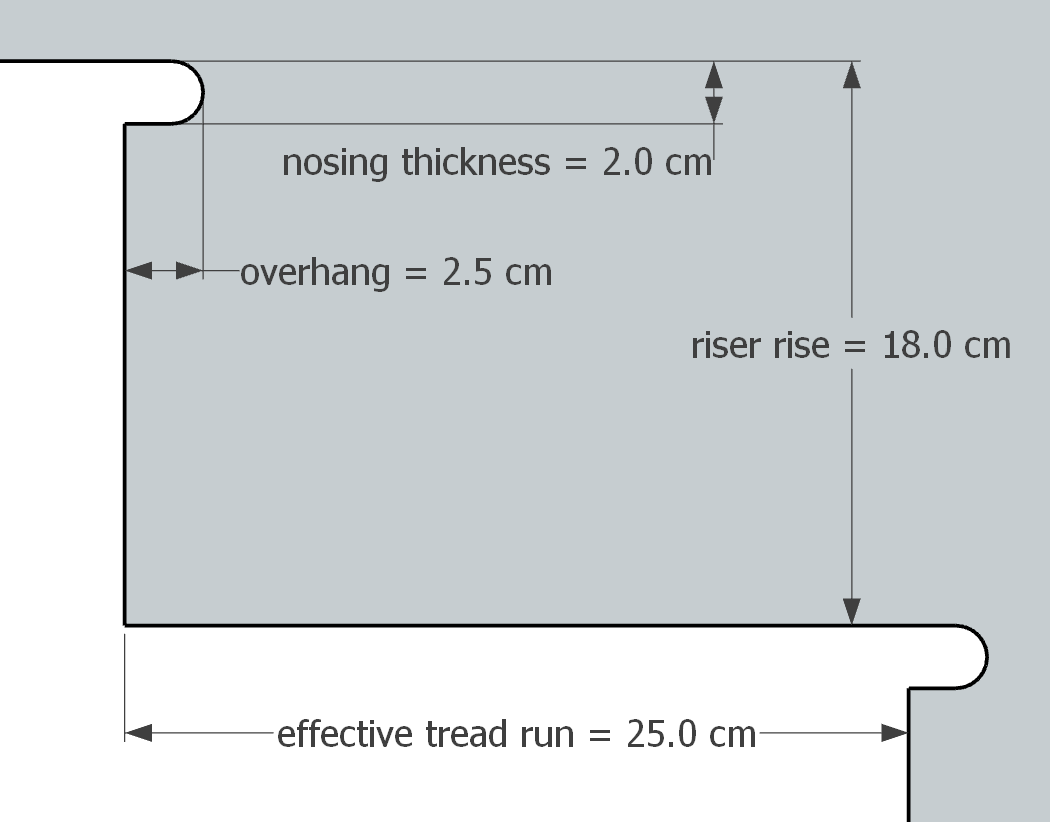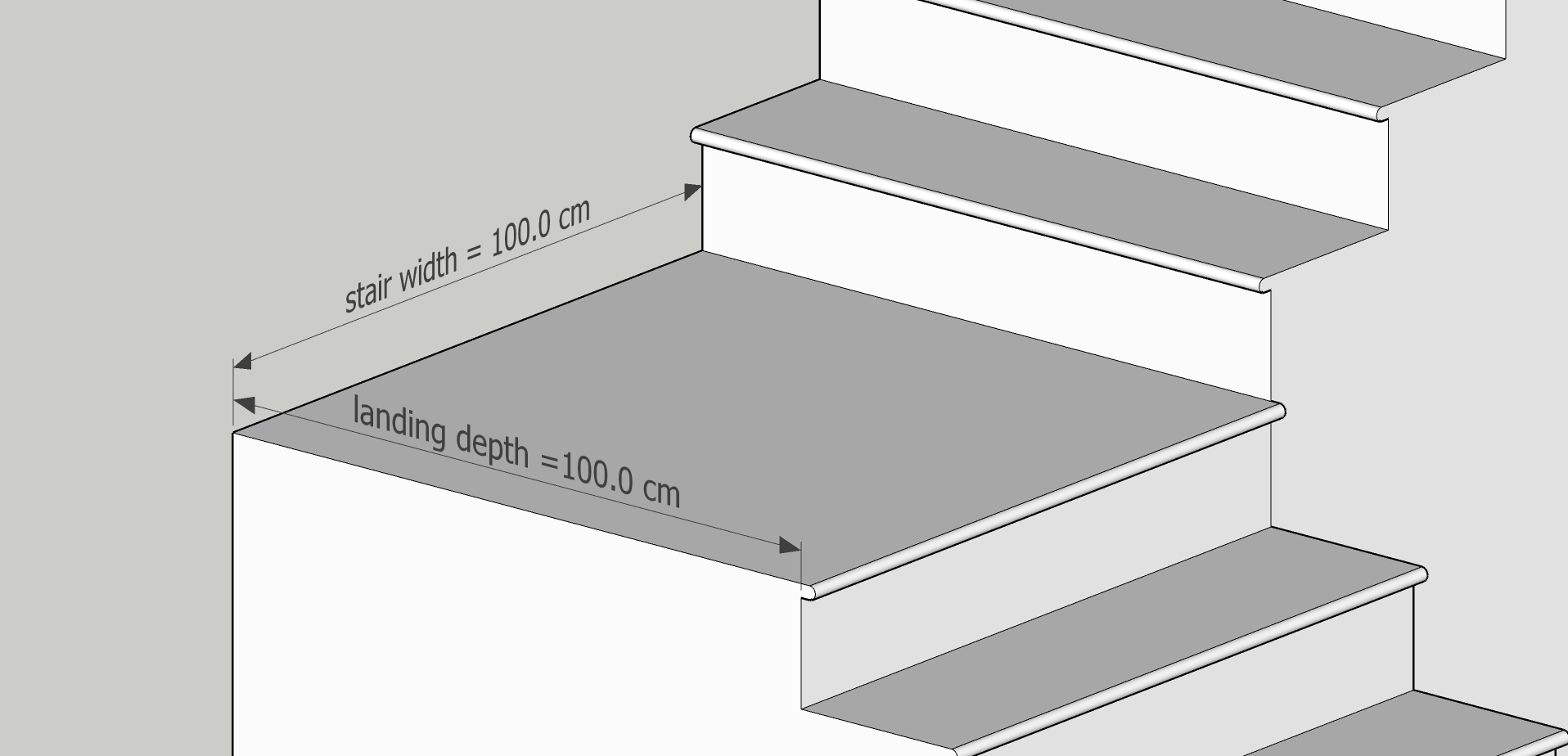# Stair Carpet Calculator

Created by Kenneth Alambra
Reviewed by Wojciech Sas, PhD candidate and Adena Benn
Last updated: Jun 19, 2022

This stair carpet calculator will help you figure out the dimensions of the carpet you will need to cover flights of stairs. Knowing precisely how much carpet you'll need will help you decide how much to purchase and could save you from spending too much. In this carpet calculator, you will learn:

• The basics of installing carpets on stairs;
• How to use this stair carpet calculator; and
• How to calculate stair carpet yourself.

As an added feature, this tool also works as a stair carpet cost calculator.

## Stair carpet installation basics

Carpeting stairs follows the same procedure as carpeting a large floor area. We use the same materials and tools like hammers and nails, staplers or tackers to affix our carpet to the surface, utility knives or hook blades to cut the carpet, and carpet knee kickers, power stretchers, or carpet tuckers to stretch and position our carpets.

The installation of stair carpets can start from the lower floor to the higher floor or landing, or vice versa. For stairs that have overhanging stair nosings, it is easier to install the carpet going up as it will be easier to tuck and staple the carpet underneath the stair nosings. On the other hand, you won't have any problems installing a carpet going down the staircase if the steps don't have overhanging nosings.

Like any flooring materials and wall claddings alike, we want to, as much as possible, have the least amount of scrap we can get after installing these materials. Other materials that we might need for the installation are tack strips, underlayment materials, and the optional double-sided adhesive tapes. In this calculator, our focus is on the amount of carpet we'll need.

## How to use this stair carpet calculator

Our stair carpet calculator is very intuitive and easy to use. Here are the steps you can follow to use this tool effectively:

1. Enter the details of your stair:

• Number of steps - we consider every floor rise as one step;
• Riser rise - the difference in elevation of or the vertical distance between two adjacent steps;
• Effective tread run - horizontal distance between same points on two adjacent steps (e.g., riser to riser measurement);
• Landing depth - if applicable, this measurement is the length of your stair landing along with the direction of the flight of stairs; and
• Stair width - the distance between the two walls on both sides of the stairs or the stair tread length.
2. If your stair nosings have overhangs, answer yes to the With stair nosing overhang? question. Answer no if they don't have.

3. If you selected yes in the second step, you would see that a Stair nosing details section will pop up within the tool. Here, you have to:

a. Select the shape of your stair nosing; and

b. Enter the measurements of the stair nosing's overhang and thickness.

4. Input the allowances you want for your carpet. You may want to have up to a foot of an allowance for the carpet length and an inch or two for the carpet width allowance. We set these allowances as part of a contingency plan if you ended up using an imprecise measuring tool or your stair has wide areas at some point of the flight.

After these steps, our calculator will display your required carpet length and width. At the same time, you will also see the carpet area if you want to estimate how much your carpet will cost. You can then use our tool as a stair carpet cost calculator by going into its Advanced mode.

Now that we know how to use our tool as a simple stair carpet calculator and a stair carpet cost calculator, how about we consider an example and learn how to calculate stair carpets manually? Let's do that in the next section of this text.

## How to determine the size of stair carpet needed

The best way to learn how to calculate stair carpet sizes is by trying to solve a problem. Let's say we first want to carpet the first portion of a staircase to look something like this:In this portion of the staircase, we need to cover a 4-step flight of stairs that has measurements of 18.0 cm for its riser rise, 25.0 cm for its effective tread run, and a landing depth of 1.0 m (or 100 cm). The steps also have 2.0 cm-thick rounded bullnose stair nosings that overhang 2.5 cm beyond the risers. It is also good to note that this flight of stairs is 1.0 m wide.

Here is a closer look at the step and nosing details:And in this illustration, you can see the landing depth and the stair width:Now, let's start by determining the length of carpet that can cover the area highlighted with green color, as shown below:As you can see, this will be the carpet section covering the riser and the nosing overhang. We can calculate the length of carpet to cover this area using this formula:

$\scriptsize V = R - t + P_\text{shape}$

where:

• $V$ - Length of carpet to cover the riser and the overhang;
• $R$ - Riser rise;
• $t$ - Nosing thickness; and
• $P_\text{shape}$ - Exposed nosing perimeter.

Since we have a rounded (or bullnose) stair nosing, we can calculate $P_\text{shape}$ using this equation:

$\scriptsize P_\text{⭘} = 2 \times (o - \frac{t}{2}) + \frac{\pi \times t}{2}$

where:

• $P_\text{⭘}$ - $P_\text{shape}$ for rounded nosing;
• $o$ - Nosing overhang; and
• $t$ - Nosing thickness.

If in case the nosing has a rectangular shape or triangular, here are the equations we could use for those, respectively:

\scriptsize \begin{align*} &P_\text{▭} = 2 \times o + t\\ &P_\text{◸} = o + \sqrt{o^2 + t^2}\\ \end{align*}

Since we have a rounded nosing, we can plug the $P_\text{⭘}$ expression into our first equation and substitute the variables with their corresponding measurements to determine $V$, as we can see here:

\scriptsize \begin{align*} V &= R - t + 2 \times (o - \frac{t}{2}) + \frac{\pi \times t}{2}\\\\ &= 18.0 - 2.0 + 2 \times (2.5 - \frac{2.0}{2}) + \frac{\pi \times2.0}{2}\\\\ &= 16.0 + 2 \times (2.5 - 1.0) + \pi\\\\ &= 16.0 + 3.0 + \pi\\\\ &= 22.1416\ \text{cm} \end{align*}

From our stair illustration, $V$ appears four times just like the number of steps there are on our flight of stairs. Consequently, there are three treads on our stairs, or a number less than the number of steps. We get the carpet length we need to cover this area by summing up these measurements. In equation form, we express that as:

$\scriptsize L = n \times V + (n - 1) \times T$

where:

• $L$ - Carpet length for a flight of stairs;
• $n$ - Number of steps on this stairs;
• $R$ - Riser rise;
• $t$ - Nosing thickness, if applicable;
• $P$ - Exposed nosing perimeter; and
• $T$ - Effective tread run.

Again, substituting our known measurements gives us:

\scriptsize \begin{align*} L &= n \times V + (n - 1) \times T\\ &= 4 \times 22.1416\ \text{cm} + (4 - 1) \times 25.0\ \text{cm}\\ &= 88.5664\ \text{cm} + (3) \times 25.0\ \text{cm}\\ &= 88.5664\ \text{cm} + 75.0\ \text{cm}\\ &= 163.5664\ \text{cm} ≈ 163.57\ \text{cm} \end{align*}

Finally, to complete the carpet length we need, we should add any carpet length we need to cover the landing of this flight of stairs. It could be the depth of a platform leading to another flight of stairs or an area before transitioning to another carpeted floor or another flooring material. Now is also the best time to consider adding an allowance for your carpet length. In equation form, we have:

$\scriptsize L_\text{total} = L + D + \text{allowance}$

where:

• $L_\text{total}$ - Total carpet length for a flight of stairs;
• $D$ - Landing depth; and
• $\text{allowance}$ - total allowance to cover any measurement errors or discrepancies.

Let's say we want to have an allowance of 10.0 cm; we now solve for the total carpet length as shown below:

\scriptsize \begin{align*} L_\text{total} &= L + D + \text{allowance}\\ &= 163.57\ \text{cm} + 100.0\ \text{cm} + 10.0\ \text{cm}\\ &= 273.57\ \text{cm} \end{align*}

For the width of our carpet, we can order a rug with the same width as our stair steps. However, to consider any deviations of the stair width throughout the staircase, perhaps due to errors in the stair's construction, it's also a good practice to consider adding an allowance to the width of the carpet. This allowance can also give you a choice to scrunch and tuck your carpet's edge against the wall or the stringers of your stairs.

Say we want a 1.0 cm allowance to both sides of our carpet for the purpose mentioned earlier, we then have to inform our supplier to provide us with a 102.0-cm wide carpet that is about 274 or 280 cm long for this flight of stairs.

💡 If you choose to install a stair runner carpet instead and wonder how to calculate carpet for stair runners, you can follow the calculation steps we discussed. But you can skip the last steps about the carpet width. Just make sure that your stair runner carpet's width is less than your stair's width.

## How to calculate the cost of stair carpet

Almost every supplier of do-it-yourself carpeting materials prices their rugs per unit area. That means it's better to know the area you need to cover with carpet if you want to calculate the cost of the stair carpet you need. So from our example earlier, we first need to calculate our needed carpet area as follows:

\scriptsize \begin{align*} \text{area} &= L_\text{total} \times W_\text{total}\\ &= 273.57\ \text{cm} \times 102.0\ \text{cm}\\ &= 27,904.14\ \text{cm}^2\\ &≈ 2.79\ \text{m}^2\\ \end{align*}

where:

• $\text{area}$ - Carpet area needed for a flight of stairs;
• $L_\text{total}$ - Total carpet length for this flight of stairs; and
• $W_\text{total}$ - Total carpet width for this flight of stairs.

Then, we multiply our calculated carpet area by the price of our carpet of choice. Let's say we got a great deal of 32 per square meter of high-quality carpet. We calculate our total carpet cost in this fashion: \scriptsize \begin{align*} \text{Carpet cost} &= \text{area} \times \text{price}\\ &= 2.7904\ \text{m}^2 \times \ 32 / \text{m}^2 \\ &= \ 89.29 \\ \end{align*} ## FAQ ### How do I calculate carpet for stair runner? To calculate carpet for stair runner for stairs with no nosing overhang: 1. Measure your stair's riser rise and tread run. Say, 10 inches and 12 inches, respectively. 2. Count how many steps your flight of stairs has. Say, 14 steps. 3. Calculate your needed carpet length by substituting these measurements in this formula: length = riser rise × number of steps + tread run × (number of steps - 1). That is length = 10 inches × 14 + 12 inches × (14 - 1) = 296 inches. ### Where do I start my stair carpet? You can start your stair carpet installation either from the lower end of your stairs or from the upper end. If it is your first time doing this yourself, you may want to start from the lower end as you may find it easier to push and tuck your carpet this way. That is because you won't be stepping over the free end of the rug while you go up, which is what happens if you start from the upper end. ### Are stairs expensive to carpet? Stair carpet cost depends on your desired carpet design, weight, and the design of your stairs. A 95-cm straight flight of stairs with a 20-cm riser rise and 25-cm tread run may cost around3.50 - $120.00 per step. However, that price range can go up after considering the other materials needed for installation and the labor cost. ### What is the cost to carpet a 12-step stairs? Covering a 12-step stair with a$20 per square meter of carpet would cost around $120 for the carpet. That holds if we consider a straight staircase with a width of 100 cm, a stair riser rise of 20 cm, an effective tread run of 25 cm, and a stair nosing overhang of around 2.5 cm. If you want to use a stair runner and leave about 7.5 cm of hardwood exposed on both sides, your carpet would cost about$100.

Kenneth Alambra
Stair details
Number of steps
steps
Riser rise
in
in
Landing depth
in
Stair width
in
With stair nosing overhang?
no
Allowances
Extra length
in
Extra width
in
Results
Carpet length
in
Carpet width
in
Carpet area
ft²
People also viewed…

### Concrete block fill

This concrete block fill calculator will help you determine how much concrete or mortar is needed to fill the void spaces inside a hollow concrete block wall of any size.

### Free fall

Our free fall calculator can find the velocity of a falling object and the height it drops from.

### Heat loss

Use the heat loss calculator to estimate the power of a heater needed to keep your room at a comfortable temperature.

### Meat footprint

Check out the meat impact - on the environment and your health.